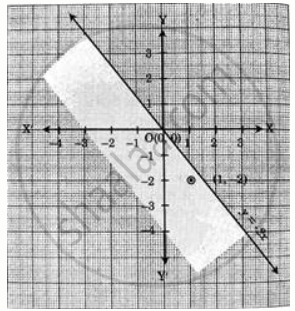# Solve the following inequations graphically in two-dimensional plane.y ≤ – 2x - Mathematics and Statistics

Graph

Solve the following inequations graphically in two-dimensional plane.
y ≤ – 2x

#### Solution

Consider the equation y = 2x.
This is the equation of a line passing through origin in third and fourth quadrant inclined.
more towards Y-axis.Consider a point (2, 1).
Substitute in equation
y ≤ – 2x
⇒ 1 ≤  2(2)
⇒ 1 ≤ –  4
shows that point (2,  1) does not satisfy the inequation.
The solution is in the other plane away from that point.

Concept: Graphical Solution of Linear Inequality of Two Variable
Is there an error in this question or solution?
Chapter 8: Linear Inequations - Exercise 8.2 [Page 120]

#### APPEARS IN

Balbharati Mathematics and Statistics 2 (Commerce) 11th Standard HSC Maharashtra State Board
Chapter 8 Linear Inequations
Exercise 8.2 | Q 1. (iii) | Page 120
Share